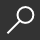{ "item_title" : "Algebra 1 Workbook", "item_author" : [" Richard Carter "], "item_description" : "LEARN AND IMPROVE YOUR ALGEBRA SKILLSThis book contains: Basic operations, number and integers, properties, rules and tipsMonomials, Binomials and Polynomials operationsHow to find Least Common Multiple and Greatest Common Factor, Factorization and Prime Numbers Different types of expressions, and related ways of solutionsDifferent types of equations, Inequalities and Functions with the related ways of solutions Many exercises the reader can do for each different argument with related explanations and solutionsAlgebra is a very noteworthy subfield of mathematics in its versatility alone if nothing else. You will be hard-pressed to find one single area of mathematics that is taught after algebra in which algebra is not practiced in almost every situation.The most general and the most commonly used definition of algebra is the study of mathematical symbols as well as the study of the manipulation of these symbols.Mathematical symbols are one of the most basic elements of mathematics, aside from numbers themselves and operation symbols, so the study of these symbols is one of the most important studies that one can take up as far as mathematics is concerned.To that end, in this book, you will find some of the most important topics regarding algebra.These include but are not limited to the following: understanding integers and basic operations, inequalities and one-step operations;fractions and factors; the main rules of arithmetic; linear equations in the coordinate plane, expressions, equations and functions; real numbers; solving linear equations; visualizing linear functions, linear equations, linear inequalities, systems of linear equations and inequalities; exponents and exponential function; polynomials, quadratic equations, radical expression, radical equations, rational expressions; and finally, intermediate topics in algebra.", "item_img_path" : "https://covers4.booksamillion.com/covers/bam/1/79/034/009/1790340098_b.jpg", "price_data" : { "retail_price" : "12.95", "online_price" : "12.95", "our_price" : "12.95", "club_price" : "12.95", "savings_pct" : "0", "savings_amt" : "0.00", "club_savings_pct" : "0", "club_savings_amt" : "0.00", "discount_pct" : "10", "store_price" : "" } }Algebra 1 Workbook : The Self-Teaching Guide and Practice Workbook with Exercises and Related Explained Solution. You Will Get and Improve
local_shippingFor DeliveryIn Stock.
This item is Non-Returnable.
FREE Shipping for Club Members help
storeBuy Online Pickup At Store### Overview

LEARN AND IMPROVE YOUR ALGEBRA SKILLS

This book contains:

• Basic operations, number and integers, properties, rules and tips
• Monomials, Binomials and Polynomials operations
• How to find Least Common Multiple and Greatest Common Factor, Factorization and Prime Numbers
• Different types of expressions, and related ways of solutions
• Different types of equations, Inequalities and Functions with the related ways of solutions
• Many exercises the reader can do for each different argument with related explanations and solutions

Algebra is a very noteworthy subfield of mathematics in its versatility alone if nothing else. You will be hard-pressed to find one single area of mathematics that is taught after algebra in which algebra is not practiced in almost every situation.

The most general and the most commonly used definition of algebra is the study of mathematical symbols as well as the study of the manipulation of these symbols.

Mathematical symbols are one of the most basic elements of mathematics, aside from numbers themselves and operation symbols, so the study of these symbols is one of the most important studies that one can take up as far as mathematics is concerned.

To that end, in this book, you will find some of the most important topics regarding algebra.

These include but are not limited to the following: understanding integers and basic operations, inequalities and one-step operations;

fractions and factors; the main rules of arithmetic; linear equations in the coordinate plane, expressions, equations and functions; real numbers; solving linear equations; visualizing linear functions, linear equations, linear inequalities, systems of linear equations and inequalities; exponents and exponential function; polynomials, quadratic equations, radical expression, radical equations, rational expressions; and finally, intermediate topics in algebra.

This item is Non-Returnable

• ISBN-13: 9781790340095
• ISBN-10: 1790340098
• Publisher: Independently Published
• Publish Date: December 2018
• Dimensions: 9.02 x 5.98 x 0.73 inches
• Shipping Weight: 1.04 pounds
• Page Count: 352